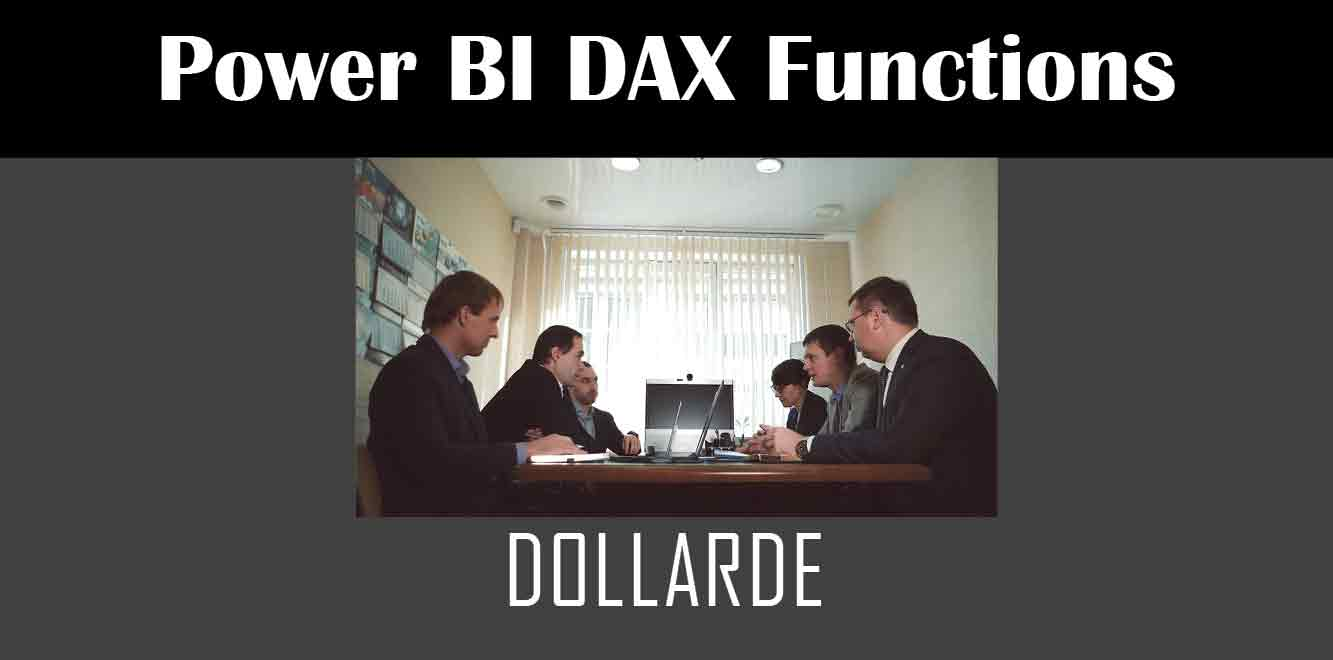# DOLLARDE

## Y## Understanding the DOLLARDE Function

The DOLLARDE function is a part of the Data Analysis Expressions (DAX) language, which is a formula language used in Power BI. The function allows you to convert a numeric value into a specific currency value.

The syntax of the DOLLARDE function is as follows:

DOLLARDE(decimal_number, fraction)

``` - decimal_number: This is a required field and represents the decimal number that needs to be converted into a currency. - fraction: This is also a required field and represents the fraction of the currency that you want to use. For example, if you want to convert a decimal number into a currency that has two decimal places, such as USD, you would use a fraction of 0.01. Using DOLLARDE Function in Power BI To use the DOLLARDE function in Power BI, follow the steps below: 1. Open the Power BI Desktop and create a new report. 2. Select the table or column that contains the decimal number that you want to convert into a currency. 3. Click on the "New Measure" button in the "Modeling" tab. 4. Enter the following DAX formula into the formula bar: ```

Currency Value = DOLLARDE(decimal_number, fraction)

``` 5. Replace the "decimal_number" and "fraction" fields with the appropriate values. 6. Press "Enter" to create the new measure. 7. Drag and drop the new measure onto the report. Examples of Using DOLLARDE Function in Power BI Here are some examples of how you can use the DOLLARDE function in Power BI: Example 1: Converting a Decimal Value to USD Suppose you have a table that contains decimal values of sales revenue, and you want to convert these values into USD. Here's how you can use the DOLLARDE function to accomplish this: ```

Sales in USD = DOLLARDE(Sales, 0.01)

``` Example 2: Converting a Decimal Value to EUR Suppose you have a table that contains decimal values of sales revenue, and you want to convert these values into EUR. Here's how you can use the DOLLARDE function to accomplish this: ```

Sales in EUR = DOLLARDE(Sales, 0.01)

``` The DOLLARDE function is a powerful tool that allows you to convert decimal values into a specific currency of your choice. By following the steps outlined in this article, you can easily use the DOLLARDE function in Power BI to convert your decimal values into the desired currency. Power BI DAX Training Courses by G Com Solutions (0800 998 9248)Power BI DAX Intensive Training Course £1,050.00 – £26,550.00 Select optionsContinue Loading DonePower BI DAX Introduction £395.00 – £9,750.00 Select optionsContinue Loading DonePower BI DAX Intermediate £395.00 – £9,750.00 Select optionsContinue Loading DonePower BI DAX Advanced £395.00 – £9,750.00 Select optionsContinue Loading Done Upcoming Courses Contact Us Subject Your Name (required) Company/Organisation Email (required) Telephone Training Course(s) Power BI Intensive TrainingPower BI introduction Power BI IntermediatePower BI AdvancedDAXPower Query MPower BI CertificationPower BI AdministrationPower PlatformPower AutomatePower AppsOTHER Your Message Upload Example Document(s) (Zip multiple files) ```
``` ```
``` ```
``` ```
``` ```
``` ```